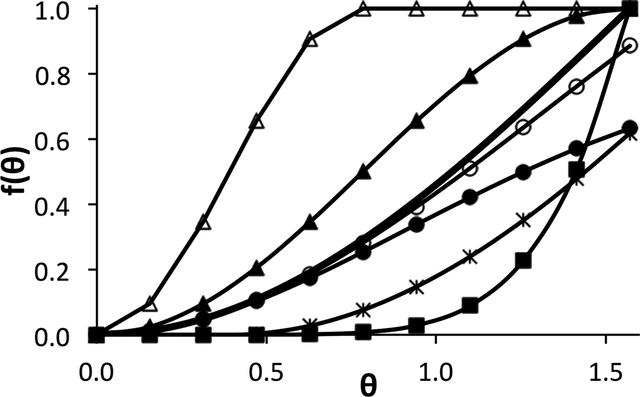disable zoom     view article Figure 1 Examples of various parallelity targets, θ0 = 0. Heavy line with no markers: f(θ) = 1 − cosθ, equation (4). Line with filled triangles: f(θ) = 1 − cos2θ, equation (8). Line with open triangles: f(θ) = 1 − cos4θ for θ ≤ π/4, equation (9). Lines with black (Ω = 1) and open (Ω = 2) circles: f(θ) = Ω2{1 − exp[(cosθ − 1)/Ω2]}, equation (7). Line with filled squares: f(θ) = (1 − cosθ)4, equation (10). Line with asterisks: f(θ), slack function, equation (11).JOURNAL OFAPPLIEDCRYSTALLOGRAPHY
ISSN: 1600-5767
Volume 48| Part 4| August 2015| Pages 1130-1141